# Measurable function

(diff) ← Older revision | Latest revision (diff) | Newer revision → (diff)

Originally, a measurable function was understood to be a functionof a real variablewith the property that for everythe setof pointsat which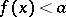is a (Lebesgue-) measurable set. A measurable function on an interval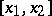can be made continuous onby changing its values on a set of arbitrarily small measure; this is the so-called-property of measurable functions (N.N. Luzin, 1913, cf. also Luzin-property).

A measurable function on a spaceis defined relative to a chosen systemof measurable sets in. Ifis a-ring, then a real-valued functiononis said to be a measurable function if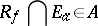for every real number, where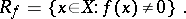This definition is equivalent to the following: A real-valued functionis measurable iffor every Borel set. Whenis a-algebra, a functionis measurable if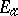(or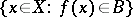) is measurable. The class of measurable functions is closed under the arithmetical and lattice operations; that is, if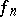,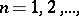are measurable, then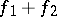,,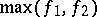,and(real) are measurable;and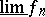are also measurable. A complex-valued function is measurable if its real and imaginary parts are measurable. A generalization of the concept of a measurable function is that of a measurable mapping from one measurable space to another.

How to Cite This Entry:
Measurable function. Encyclopedia of Mathematics. URL: http://encyclopediaofmath.org/index.php?title=Measurable_function&oldid=11460
This article was adapted from an original article by V.V. Sazonov (originator), which appeared in Encyclopedia of Mathematics - ISBN 1402006098. See original article